## Filters

Sort by :
Clear All
Q

Q 4    Here is an incomplete net for making a cube. Complete it in at least two different
ways. Remember that a cube has six faces. How many are there in the net here?
(Give two separate diagrams. If you like, you may use a squared sheet for easy
manipulation.)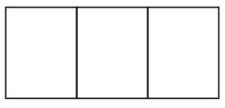There are three faces in the given net. The two possibilities of forming nets are

Q 2     Dice are cubes with dots on each face. Opposite faces of a die always have a total of seven dots on them. Here are two nets to make dice (cubes); the numbers inserted in each square indicate
the number of dots in that box.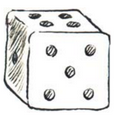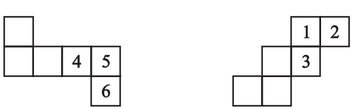Insert suitable numbers in the blanks, remembering that the number on the
opposite faces should total to 7.

The sum of the opposite sides of a dice =7

Draw a view of each solid as seen from the direction indicated by the arrow.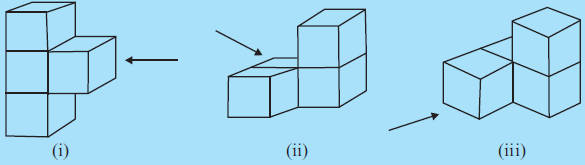Drawn view of each solid as seen from the direction indicated by the arrow are  : -   i )    ii )         iii )

For each solid, the three views (1), (2), (3) are given. Identify for each solid the corresponding top, front and side views.For a ) For b) For c) For d)

Q. 3.     Examine if the following are true statements:

(i) The cube can cast a shadow in the shape of a rectangle.
(ii) The cube can cast a shadow in the shape of a hexagon.

A cube can cast shadow only in the shape of a square. Therefore,  both statements (i) and (ii) are not possible

Q. 1.     What cross-sections do you get when you give a

(i) vertical cut          (ii) horizontal cut

to the following solids?

(a) A brick        (b) A round apple          (c) A die     (d) A circular pipe        (e) An ice cream cone

a )  A brick i ) Vertical cut  A vertical cut gives a square or rectangular crossection ii )  The horizontal cut  gives rectangular crossection b ) A round apple  In both, the case crossection will be circular in shape approximately c ) A die both the cut will give a square shape   d ) A circular pipe  i ) A vertical cut will give a circular shape ii ) The horizontal cut ...

Three cubes each with 2 cm edge are placed side by side to form a cuboid. Try to make an oblique sketch  and say what could be its length, breadth and height.

Oblique sketch of three cubes each with 2 cm edge  placed side by side to each other to form a cuboid is  In this case  lenght = 2+2+2= 6cm breath = 2 cm height = 2cm Two more cases are possible with Height = 2+2+2= 6cm breath = 2 cm length = 2cm And  breath = 2+2+2= 6cm length = 2 cm height = 2cm

Two dice are placed side by side as shown: Can you say what the total would be on the face opposite to
(a) 5 + 6          (b) 4 + 3

(Remember that in a die sum of numbers on opposite faces is 7)

a )  Face opposite to 5 is  2 and opposite to 6  is 1 Therefore, there sum is equal to = 2 + 1 = 3 b )  Face opposite to 4 is 3 and opposite to 3 is 4 Therefore, there sum is equal to = 3 + 4 = 7

Try to guess the number of cubes in the following arrangements (Fig 15.17).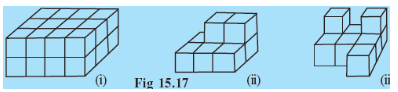i) There are 4 columns and 2 rows in the first line. So, the total  number of cubes in the first line is    And there is total 3 such line. Therefore, the total number of cubes are  ii) There are 3 columns and 1 row in the first line at the bottom. So, the total number of cubes in the first line are And there are total 2 such lines  So, the total number of cubes at the bottom...

Q. 5.     Give (i) an oblique sketch and (ii) an isometric sketch for each of the following:

(a) A cuboid of dimensions 5 cm, 3 cm and 2 cm. (Is your sketch unique?)
(b) A cube with an edge 4 cm long.
An isometric sheet is attached at the end of the book. You could try to make on it some cubes or cuboids of  dimensions specified by your friend.

The isometric sketch of  s cuboid of dimensions 5 cm, 3 cm and 2 cm. In a similar way, we can draw for the cube also

Q. 4.     Make an oblique sketch for each one of the given isometric shapes: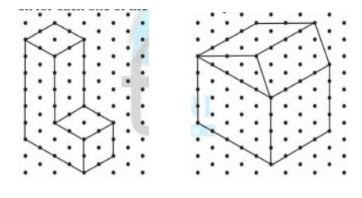Oblique sketch for each one of the given isometric shapes is: -

Q. 3.     Three cubes each with 2 cm edge are placed side by side to form a cuboid. Sketch an oblique or isometric sketch of this cuboid.

It is given that three cubes each with 2 cm edge are placed side by side to form a cuboid So, the oblique sketch of the cuboid is given below

Q. 2.     The dimensions of a cuboid are 5 cm, 3 cm and 2 cm. Draw three different isometric sketches of this cuboid.

There are a total of 3 isometric sketches of the cuboid are possible with given dimensions of a cuboid

Q. 5.     Match the nets with appropriate solids: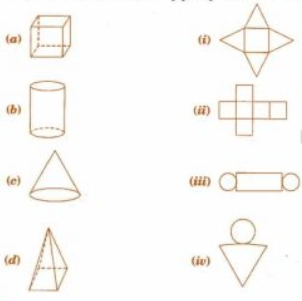Thsese are the correct matches

Q. 3.     Can this be a net for a die? Explain your answer.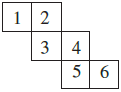NO,  This can not be a net for a die because the opposite faces of a die always have a total sum of 7. Now, this case one pair is  4  opposite to 1 So, their sum is equal to  5  and not equal to 7 And also, the 2nd pair is 6  opposite to 3 . So, their sum is equal to 9 and not equal to 7

Q.1     Here you find four nets (Fig 15.10). There are two correct nets among them to make a tetrahedron. See if you can work out which nets will make a tetrahedron.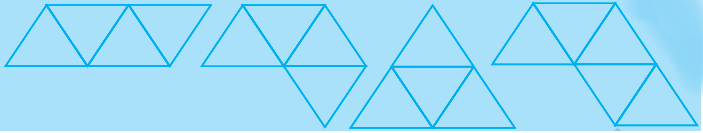Out of these four nets, we can make a  tetrahedron with net (i) and (iii)

Complete the following table: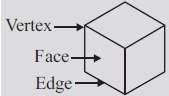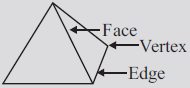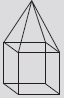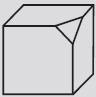Faces (F) 6 4 Edges (E) 12 Vertices (V) 8 4

Faces(F) 6 4 10 7 Edges(E) 12 6 16 15 Vertices(V) 8 4 9 10

Q. 1     Match the shape with the name: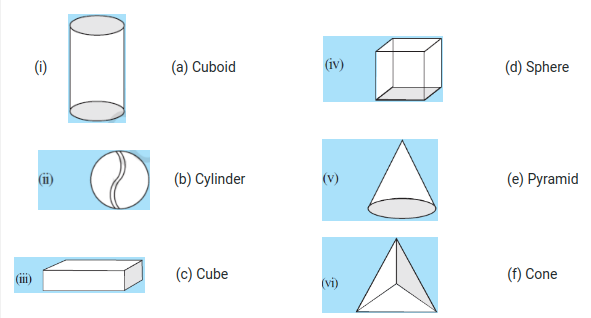These are the correct matches
Exams
Articles
Questions